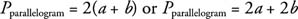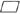## Parallelograms

In the parallelogram shown in Figure 1h is a height because it is perpendicular to a pair of opposite sides called bases. One of the bases has been labeled b, and the nonbase remaining sides are each labeled a.Figure 1 A parallelogram with base and height labeled.

## Finding the perimeter

The following formula is now apparent.## Finding the Area

In Figure , also notice that Δ WXV ≅ Δ TYZ, which means that they also have equal areas. This makes the area ofWXYT the same as the area ofXYZV. But A rectangle XYZV = bh, so A parallelogram XYTW = bh.That is, the area of a parallelogram is the product of any base with its respective height.Example 1: Find the perimeter and area of Figure 2.Figure 2 Finding the perimeter and area of a parallelogram.

The figure is a parallelogram, so